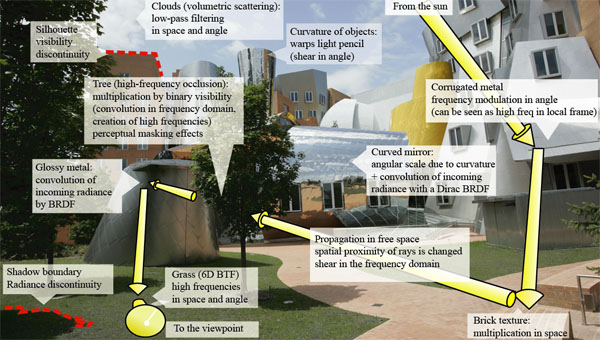# A Frequency analysis of Light Transport

## MIT CSAIL and ARTIS-GRAVIR/INRIA-IMAG

Transactions on Graphics, Proceedings of SIGGRAPH 2005## Abstract

We present a signal-processing framework for light transport. We study the frequency content of radiance and how it is affected by phenomena such as shading, occlusion, and travel in free space. This extends previous work that considered either spatial or angular dimensions, and offers a comprehensive treatment of both space and angle.

We characterize how the radiance signal is modified as light propagates and interacts with objects. In particular, we show that occlusion (a multiplication in the primal space) amounts in the Fourier domain to a convolution by the frequency content of the blocker. Propagation in free space corresponds to a shear in the space-angle frequency domain, while reflection on curved objects performs a different shear along the angular frequency axis. As described by previous work, reflection is a convolution in the primal space, and therefore amounts to a multiplication in the Fourier domain. Our extension shows how the spatial components of lighting are affected by this angular convolution.

We show that our signal-processing framework predicts the characteristics of interactions such as caustics, and the disappearance of the shadows of small features. Predictions on the frequency spectrum of the radiance function can then be used to control sampling rates or the choice of reconstruction kernels for rendering. Other potential applications include pre-computed radiance transfer and inverse rendering.

PDF(15MB)

Video (70MB)

Slides early version (ppt), Siggraph version (pdf), longer version (ppt, pdf-1, pdf-6)

## Acknowledgments

We thank Jaakko Lehtinen, the reviewers of the Artis and MIT teams, as well as the SIGGRAPH reviewers for insightful feedback.

This work was supported by an NSF CAREER award 0447561 Transient Signal Processing for Realistic Imagery, an NSF CISE Research Infrastructure Award (EIA9802220), an ASEE National Defense Science and Engineering Graduate fellowship, the European Union IST-2001-34744 RealReflect project, an INRIA équipe associée, and the MIT-France program.

## FAQ

##### Why 4D and not 5D?

We need to keep the fifth dimension as "time" or propagation.
However, the study of participating media would probably require a full 5D treatment.

The gradient can be expressed in the Fourier domain as a multiplication by i omega. This means that the gradient emphasizes the high frequencies.

##### Why not use wavelets?

Wavelets are great for actual computation, but not as good for theoretical analysis. The convolution theorem does not apply to wavelets. We emphasize again that we do not advocate the use of Fourier bases for computation (our proof of concept had not sine or cosine!), we only recommend the use of Fourier transform for bandwidth analysis.

##### How do I use it for PRT?

Pretty much the same criterion we describe for ray tracing applies to PRT. The spatial sampling of PRT should depends on the bandwidth of the incoming light, the BRDF, the curvature and the inverse distance to blockers. This (correctly) predicts infinite bandwidth at contact points, so some clamping needs to happen.

##### How is the curvature handled in the proof of concept application?

The way we compute curvature is based on a normal buffer. We first render the primary rays and extract both a depth buffer and a normal buffer. We then do a finite difference computation of curvature from this map, which encapsulates both the curvature and the incidence angle term (see the C++ code for more detail).
As a result, it does not matter where the normals come from, whether it's real geometry or normal maps. (you could do the same by taking the curvature in the normal map if you object to rendering a high res version of the image).

##### Does the theory say anything about variance for Monte Carlo integration

The variance of a function is equal to the square integral of its Fourier spectrum for all frequency except the DC (per Parseval's theorem). Therefore, the spectrum can provide an estimation of the variance of a Monte Carlo estimator.